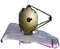# Solar System Targets Position Level 1The Solar System Targets form is used to specify moving targets in some JWST observations.  This article provides a description of the Level 1 specifications needed for the Astronomer’s Proposal Tool (APT).

Format definitions...

Purple text indicates the parameter is Limited Access.

Boldface italics type indicates the name of an APT parameter or a value for a parameter.Red text indicates restrictions on a parameter.Black text indicates an important note.

Brown text indicates notes for the developers.

Green text indicates the name of the parameter used by Commanding.

Items in brackets - <value> - are required values.

Items in square brackets - [<value>] - are optional.

For the Level 1 position, specify your target in one of the following ways:

1. STD = <object name>, where the name must be from the list of Solar System Standard Targets, or
2. TYPE = <name>.

The TYPE = <name> target description allows the specification of non-standard targets in a variety of formats and must be the first entry in the field if it is used.

Positional Parameters for TYPE = COMET

ParameterDefinition

Q = <value>

Perihelion distance, in AU

E= <value>

Eccentricity

I =<value>

Inclination, in degrees

O= <value>

Longitude of ascending node, in degrees

W = <value>

Argument of perihelion, in degrees

T = <value>

Time of perihelion passage, in TDB, TDT, or UTC

EQUINOX = <value>

either B1950, or J2000

EPOCH = <value>

Osculation date, in TDB, TDT, or UTC

[A1 = <value>]

Radial component of non-gravitational acceleration (AU/day2)

[A2 = <value>]

Component of non-gravitational acceleration lying in the orbital plane and parallel to the instantaneous velocity vector (AU/day2)

[A3 = <value>]

Component of non-gravitational acceleration directed perpendicular to the plane defined by A1 and A2 (AU/day2)

SOURCE = <value>A comment describing the source of the orbital elements.

Positional Parameters for TYPE = ASTEROID

ParameterDefinition

A = <value>

Semi-major axis, in AU

E = <value>

Eccentricity

I = <value>

Inclination, in degrees

O = <value>

Longitude of ascending node, in degrees

W = <value>

Argument of perihelion, in degrees

M = <value>

Mean anomaly at EPOCH, in degrees

EQUINOX = <value>

J2000

EPOCH = <value>

Osculation date, in TDB, TDT, or UTC

SOURCE = <value>A comment describing the source of the orbital elements.

For all cases, the required input data are described below. If the data are valid only over a specific period of time, then specify this time interval using the Solar System special requirements .It is the responsibility of the observer to supply accurate orbital elements to STScI when specifying TYPE=COMET or TYPE=ASTEROID. Note that the requirement on how fast JWST can track a moving target is 30 milliarcsec/second.

Change log